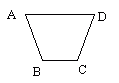Name: ___________________Date:___________________

 Email us to get an instant 20% discount on highly effective K-12 Math & English kwizNET Programs!

### Grade 3 - Mathematics8.7 Angles

 When two rays meet at a point, an angle is formed. The point at which they meet is called the vertex. An angle that measures 90 degrees is called a right angle. An angle that measures less than 90 degrees is called acute angle. An angle that measures more than 90 degrees is called obtuse angle. Squares and rectangles are made up of four right angles. Directions: Answer the following questions. Also draw right angle, acute angle, obtuse angle and name the rays, angles and vertex.
 Q 1: What is an angle that forms a square corner.abtuserightacute Q 2: These polygons has _____number of right angles.432 Q 3: This polygon has _____angles243 Q 4: Squares and Rectangles have 4 right angles.FalseTrue Question 5: This question is available to subscribers only! Question 6: This question is available to subscribers only!